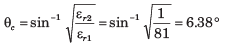Courses

# Electromagnetic Wave Propagation - MCQ Test

## 20 Questions MCQ Test GATE ECE (Electronics) 2022 Mock Test Series | Electromagnetic Wave Propagation - MCQ Test

Description
This mock test of Electromagnetic Wave Propagation - MCQ Test for Railways helps you for every Railways entrance exam. This contains 20 Multiple Choice Questions for Railways Electromagnetic Wave Propagation - MCQ Test (mcq) to study with solutions a complete question bank. The solved questions answers in this Electromagnetic Wave Propagation - MCQ Test quiz give you a good mix of easy questions and tough questions. Railways students definitely take this Electromagnetic Wave Propagation - MCQ Test exercise for a better result in the exam. You can find other Electromagnetic Wave Propagation - MCQ Test extra questions, long questions & short questions for Railways on EduRev as well by searching above.
QUESTION: 1

### At 50 MHz a lossy dielectric material is characterized by μ = 2.1μo ,ε = 3.6 εo and σ = 0.08 S/m. The electric field is Es = 6e-jγx uz V/m Que: The propagation constant γ is

Solution: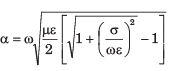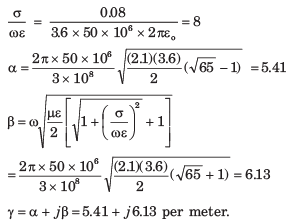QUESTION: 2

### At 50 MHz a lossy dielectric material is characterized by μ = 2.1μo ,ε = 3.6 εo and σ = 0.08 S/m. The electric field is Es = 6e-jγx uz V/m Que: The impedance η is

Solution: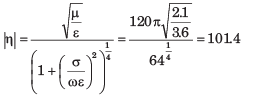QUESTION: 3

### A non magnetic medium has an intrinsic impedance 360∠30o Ω Que: The loss tangent is

Solution: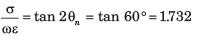QUESTION: 4

A non magnetic medium has an intrinsic impedance 360∠30o Ω

Que: The Dielectric constant is

Solution: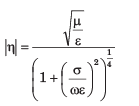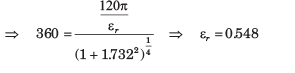QUESTION: 5

The amplitude of a wave traveling through a lossy nonmagnetic medium reduces by 18% every meter. The wave operates at 10 MHz and the electric field leads the magnetic field by 24o

Que: The propagation constant is

Solution: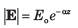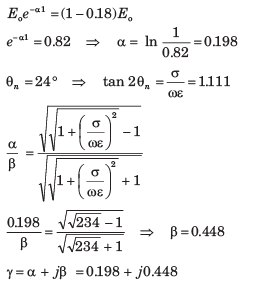QUESTION: 6

The amplitude of a wave traveling through a lossy nonmagnetic medium reduces by 18% every meter. The wave operates at 10 MHz and the electric field leads the magnetic field by 24o

Que: The skin depth is

Solution: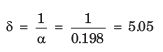QUESTION: 7

A 60 m long aluminium (σ = 3.5 x 107 S/m, μr = 1, ε2 = 1) pipe with inner and outer radii 9 mm and 12 mm carries a total current of 16 sin(106 πt)A. The effective resistance of the pipe is

Solution: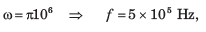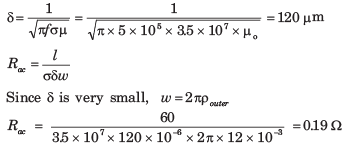QUESTION: 8

Silver plated brass wave guide is operating at 12 GHz. If at least the thickness of silver ( σ = 6.1 x 107 S/m, μr = εr = 1 ) is 5 δ the minimum thickness required for wave-guide is

Solution: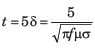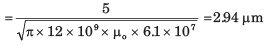QUESTION: 9

A uniform plane wave in a lossy nonmagnetic media has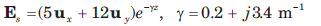The magnitude of the wave at z = 4 m and t = T/8 is

Solution: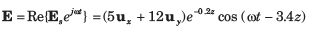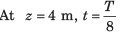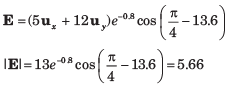QUESTION: 10

A uniform plane wave in a lossy nonmagnetic media hasQue: The loss suffered by the wave in the interval 0 < z < 3 m is

Solution: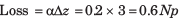1 Np =  8.686 DB, 0.6 Np = 5.21 dB.

QUESTION: 11

Region 1, z < 0 and region 2, z > 0, are both perfect dielectrics. A uniform plane wave traveling in the uz direction has a frequency of 3 x 1010 rad /s. Its wavelength in the two region are  λ1 = 5 cm and  λ2 = 3 cm.

Que: On the boundary the reflected energy is

Solution: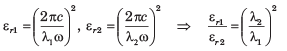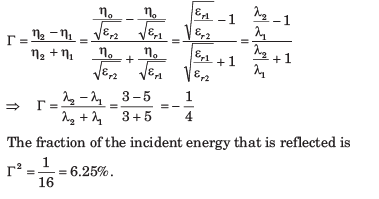QUESTION: 12

Region 1, z < 0 and region 2, z > 0, are both perfect dielectrics. A uniform plane wave traveling in the uz direction has a frequency of 3 x 1010 rad /s. Its wavelength in the two region are  λ1 = 5 cm and  λ2 = 3 cm.

Que: The SWR is

Solution: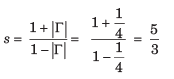QUESTION: 13

A uniform plane wave is incident from region 1 ( μr = 1, σ = 0) to free space. If the amplitude of incident wave is one-half that of reflected wave in region, then the value of εr is

Solution: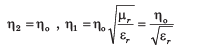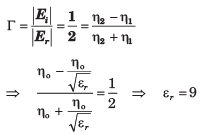QUESTION: 14

A 150 MHz uniform plane wave is normally incident from air onto a material. Measurements yield a SWR of 3 and the appearance of an electric field minimum at 0.3λ in front of the interface. The impedance of material is

Solution: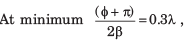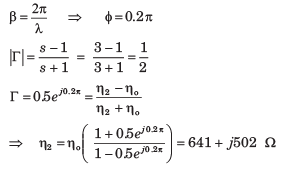QUESTION: 15

A plane wave is normally incident from air onto a semi-infinite slab of perfect dielectric (εr = 3.45) . The fraction of transmitted power is

Solution: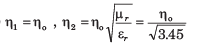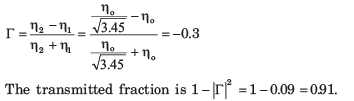QUESTION: 16

Consider three lossless region :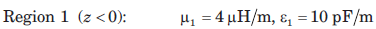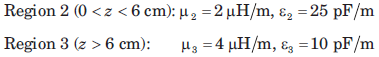Que: The lowest frequency, at which a uniform plane wave incident from region 1 onto the boundary at z = 0 will have no reflection, is

Solution:

This frequency gives the condition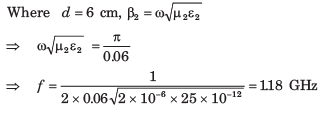QUESTION: 17

Consider three lossless region :Que: If frequency is 50 MHz, the SWR in region 1 is

Solution:

At 50 MHz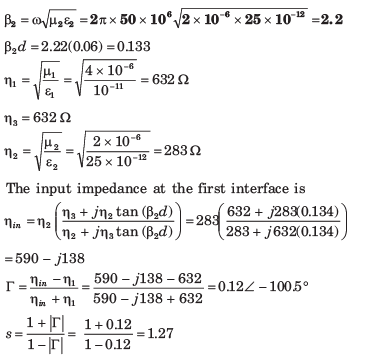QUESTION: 18

A uniform plane wave in air is normally incident onto a lossless dielectric plate of thickness  λ/8 , and of intrinsic impedance  η = 260 Ω. The SWR in front of the plate is

Solution: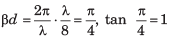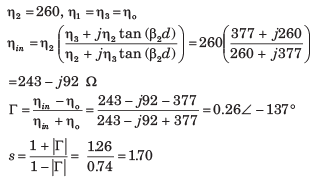QUESTION: 19

The E-field of a uniform plane wave propagating in a dielectric medium is given by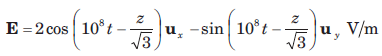The dielectric constant of medium is

Solution: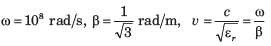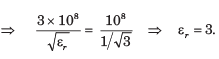QUESTION: 20

An electromagnetic wave from an under water source with perpendicular polarization is incident on a water-air interface at angle 20o with normal to surface. For water assume εr = 81, μr = 1. The critical angle θc is

Solution: